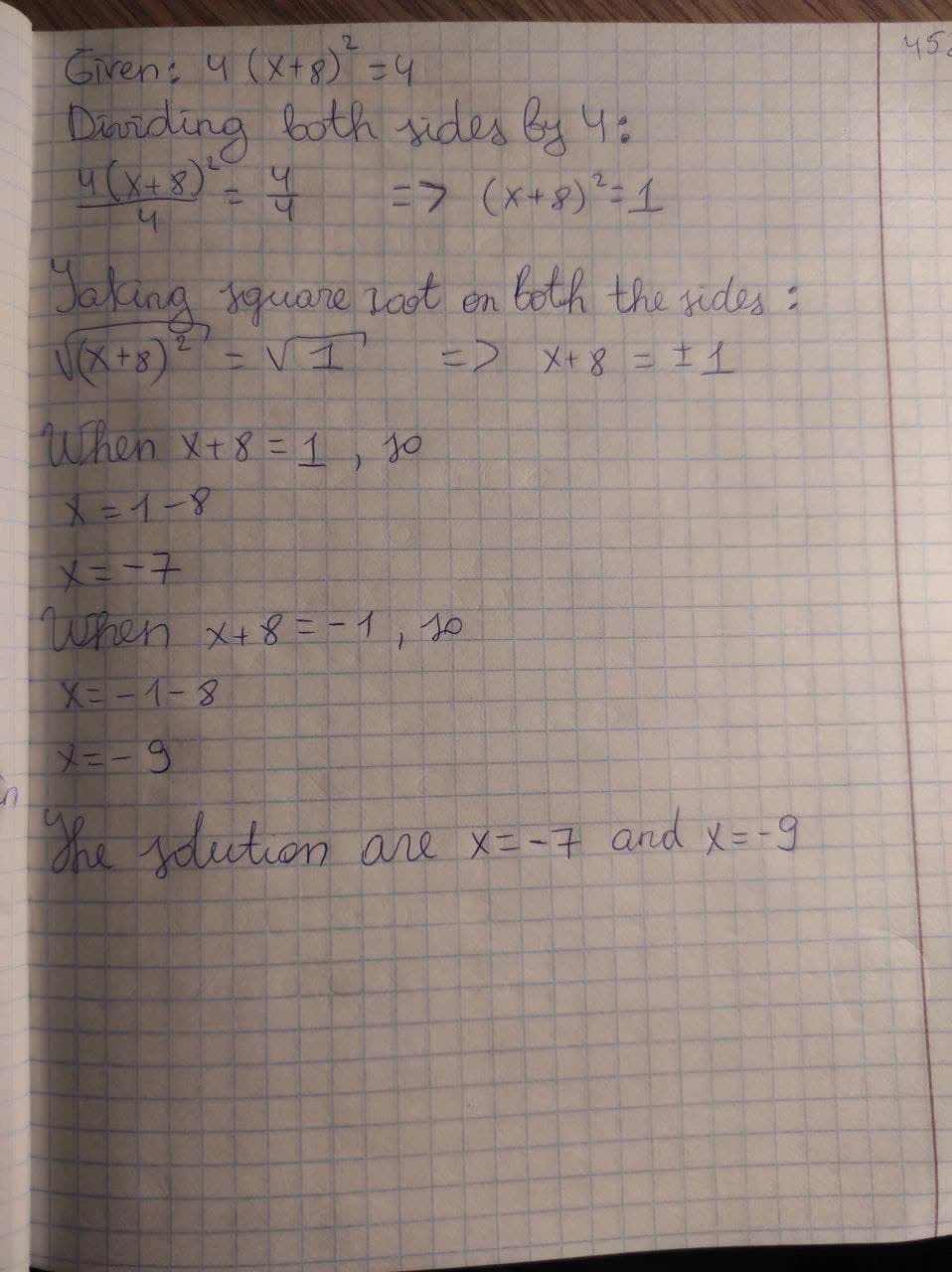# Solve the quadratic equation for all values of x in simpls form 4(x+8)^2=4Tazmin Horton 2021-03-05 Answered
Solve the quadratic equation for all values of x in simpls form
$$\displaystyle{4}{\left({x}+{8}\right)}^{{2}}={4}$$

• Questions are typically answered in as fast as 30 minutes

### Plainmath recommends

• Get a detailed answer even on the hardest topics.
• Ask an expert for a step-by-step guidance to learn to do it yourself.pattererX

Dividing ot sides by 4
$$\displaystyle\frac{{{4}{\left({x}+{8}\right)}^{{2}}}}{{4}}=\frac{{4}}{{4}}$$
$$\displaystyle{\left({x}+{8}\right)}^{{2}}={1}$$
Taking square root on both sides
$$\displaystyle\sqrt{{{\left({x}+{8}\right)}^{{2}}}}=\sqrt{{1}}$$
$$x+8=+-1$$
When $$x+8=1$$
so, $$x=1-8$$
$$x=-7$$
When $$x+8=-1$$
so, $$x=-1-8$$
$$x=-9$$
The solution are $$x=-7$$ and $$x=-9$$